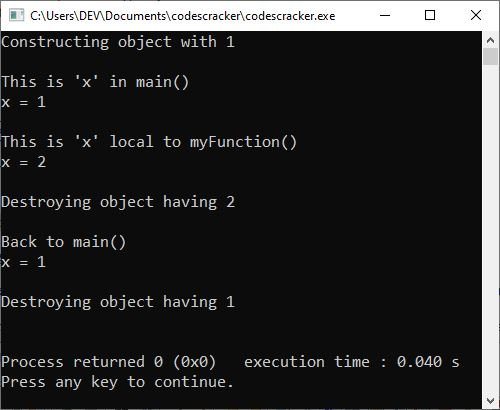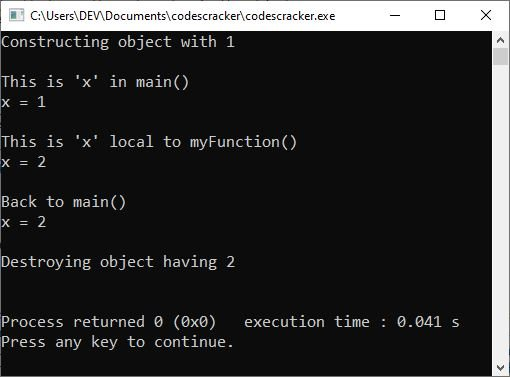# C++ Objects as Function Arguments with Examples

Objects are passed to functions in the same way that any other type of variable is passed. That is, objects may be passed both ways: through call-by-value or through call-by-reference mechanisms. Let's discuss these mechanisms.

## C++ Object Passing via Call-by-Value

When it is said that objects are passed through the call-by-value mechanism, it means that the called function creates a copy of the passed object. However, the fact that a copy is created means, in essence, that another object is created. This raises two related questions.

• Is the object's constructor executed when the copy of the passed object is made ?
• Is the destructor executed when the copy is destroyed ?

Let's take a look at the following C++ program to find out the answers to the above questions:

```#include <iostream>
using namespace std;

class myClass
{
int x;

public:
myClass(int i)          // constructor
{
x = i;
cout << "Constructing object with " << i << "\n\n";
}
~myClass()              // destructor
{
cout << "Destroying object having " << x << "\n\n";
}
void put_x(int i)
{
x = i;
}
int get_x(void)
{
return x;
}
};

void myFunction(myClass mcOb)
{
mcOb.put_x(2);              // change the value of x in the object
cout << "This is 'x' local to myFunction()\n";
cout << "x = " << mcOb.get_x() << "\n\n";
}

int main()
{
myClass S(1);               // create an object with the value 1
cout << "This is 'x' in main()\n";
cout << "x = " << S.get_x() << "\n\n";
myFunction(S);              // pass object S by value to myFunction()
cout << "Back to main()\n";
cout << "x = " << S.get_x() << "\n\n";
return 0;
}```

When the above C++ program is compiled and executed, it will produce the following output:Notice that two calls to the destructor function are executed, but only one call is made to the constructor function. As it is clear from the output, the constructor function is not called when the copy of S is created by myFunction(). The reason for this is that when an object is passed to a function (through the call-by-value mechanism), the current state of the object is passed. If the called function invokes the constructor, initialization (of the object) will occur, possibly changing the object. Thus, the constructor function is not involved when a function receives an object as its parameter; rather, it copies down the members of the passed object for the called function, and that serves as a copy of the passed object.

Note: A called function receiving an object as a parameter creates a copy of the object without invoking the constructor. However, when the function terminates, it destroys this copy of the object by invoking its destructor function.

However, it is very much necessary to destroy the copy of the object that the called function was working with before returning from the function. Thus, the destructor function must be executed when this copy is destroyed.

## C++: Passing Objects Through References

When an object is passed by value to a function, a copy of that object is created without invoking its constructor, as you may know. When the function exits, the destructor of the copy is called.

You can pass the reference to the object if you want the called function to work with the original object rather than creating and destroying a copy of it. The called function then uses the original object's reference or alias to refer to it. The following program exemplifies this:

```#include <iostream>
using namespace std;

class myClass
{
int x;

public:
myClass(int i)          // constructor
{
x = i;
cout << "Constructing object with " << i << "\n\n";
}
~myClass()              // destructor
{
cout << "Destroying object having " << x << "\n\n";
}
void put_x(int i)
{
x = i;
}
int get_x(void)
{
return x;
}
};

void myFunction(myClass &mcOb)
{
mcOb.put_x(2);              // change the value of x in the object
cout << "This is 'x' local to myFunction()\n";
cout << "x = " << mcOb.get_x() << "\n\n";
}

int main()
{
myClass S(1);               // create an object with the value 1
cout << "This is 'x' in main()\n";
cout << "x = " << S.get_x() << "\n\n";
myFunction(S);              // pass object S by value to myFunction()
cout << "Back to main()\n";
cout << "x = " << S.get_x() << "\n\n";
return 0;
}```

When the C++ program above is compiled and run, the following will happen:Now compare this output with the output of the previous program. Contrary to the previous output, here only one call has been made to the constructor function and one call to the destructor function. That is because when the object is passed to the function by reference, the formal parameter (mcOb here) of myFunction() becomes the reference (alias) of the actual parameter (S here). That means the called function myFunction() refers to the original object with an alias name; it does not make a copy of the passed object. Thus, no constructor function is invoked since no new object has been created.

Furthermore, when the function myFunction() is terminated, no destructor is invoked because there is no copy of the object that is to be destroyed that is exclusive to myFunction().

However, the constructor is invoked when the object S is declared by the statement myClass S(1), and the destructor is invoked when this object S is destroyed, that is, when main() terminates. When passing parameters by reference, remember that changes to the object inside the function affect the calling object.

C++ Quiz

« Previous Tutorial Next Tutorial »